SLVS845G March   2009  – August 2014

PRODUCTION DATA.

1. Features
2. Applications
3. Description
4. Revision History
5. Pin Configuration and Functions
6. Specifications
7. Detailed Description
1. 7.1 Overview
2. 7.2 Functional Block Diagram
3. 7.3 Feature Description
4. 7.4 Device Functional Modes
8. Application and Implementation
1. 8.1 Application Information
2. 8.2 Typical Application
1. 8.2.1 Design Requirements
2. 8.2.2 Detailed Design Procedure
3. 8.2.3 Application Curves
9. Power Supply Recommendations
10. 10Layout
1. 10.1 Layout Guidelines
2. 10.2 Layout Example
11. 11Device and Documentation Support
12. 12Mechanical, Packaging, and Orderable Information

• PWP|20
• PWP|20

## 6 Specifications

### 6.1 Absolute Maximum Ratings

over operating free-air temperature (unless otherwise noted) (1)
MIN MAX UNIT
Input voltage EN –0.3 60 V
VIN –0.3 60
VReg –0.3 20
LPM –0.3 5.5
OV_TH –0.3 5.5
RST_TH –0.3 5.5
SYNC –0.3 5.5
VSENSE –0.3 5.5
Output voltage BOOT –0.3 65 V
PH –0.3 60
30 ns –2 60
200 ns –1 60
TJ = –40 –0.85 60
TJ = 125 –0.5 60
RT –0.3 5.5 V
RST –0.3 5.5
Rslew –0.3 5.5
Cdly –0.3 8
SS –0.3 8
COMP –0.3 7
TJ Operating virtual junction temperature range –40 150 °C
(1) Stresses beyond those listed under Absolute Maximum Ratings may cause permanent damage to the device. These are stress ratings only, which do not imply functional operation of the device at these or any other conditions beyond those indicated under Recommended Operating Conditions. Exposure to absolute-maximum-rated conditions for extended periods may affect device reliability.

### 6.2 Handling Ratings

MIN MAX UNIT
Tstg Storage temperature range –55 165 °C
V(ESD) Electrostatic discharge Human-body model (HBM), per AEC Q100-002(1) –2 2 kV
Charged-device model (CDM), per AEC Q100-011 –750 750 V
(1) AEC Q100-002 indicates HBM stressing is done in accordance with the ANSI/ESDA/JEDEC JS-001 specification.

### 6.3 Recommended Operating Conditions

over operating free-air temperature (unless otherwise noted)
MIN MAX UNIT
VI Unregulated buck supply input voltage (VIN, EN) 3.6 48 V
V(VReg) Regulator voltage range 0.9 18 V
Power up in low-power mode (LPM) or discontinuous mode (DCM) 0.9 5.5
Logic level inputs (RST, VSENSE, OV_TH, RST_TH, SYNC, RT) 0 5.25 V
Logic level inputs (SS, Cdly, COMP) 0 6.5 V
TJ Operating junction temperature range(1) –40 150 °C
(1) This assumes TA = TJ – Power dissipation × RθJA (junction-to-ambient).

### 6.4 Thermal Information

THERMAL METRIC(1) TPS54362-Q1 UNIT
PWP
20 PINS
RθJA Junction-to-ambient thermal resistance 43.8 °C/W
RθJC(top) Junction-to-case (top) thermal resistance 21.4
RθJB Junction-to-board thermal resistance 18.5
ψJT Junction-to-top characterization parameter 0.5
ψJB Junction-to-board characterization parameter 18.3
RθJC(bot) Junction-to-case (bottom) thermal resistance 1.2

### 6.5 Electrical Characteristics

V(VIN) = 7 V to 48 V, V(EN) = V(VIN), TJ = –40°C to 150°C (unless otherwise noted)
PARAMETER TEST CONDITIONS MIN TYP MAX UNIT TEST(1)
INPUT POWER SUPPLY
V(VIN) Supply voltage on VIN pin Normal mode–buck mode after initial start-up 3.6 48 V Info
Low-power mode Falling threshold (LPM disabled) 8 V
Rising threshold (LPM activated) 8.5
High-voltage threshold (LPM disabled) 25 27 30
I(q-Normal) Quiescent current, normal mode Open-loop test – maximum duty cycle
V(VIN) = 7 V to 48 V
5 10 mA PT
I(q-LPM) Quiescent current; low-power mode I(VReg) < 1 mA, V(VIN) = 12 V, TA = 25°C 65 75 μA PT
I(VReg) < 1 mA, V(VIN) = 12 V, –40 < TJ < 150°C 75
I(VReg) < 1 mA, V(VIN) = 24 V, TA = 25°C 85
I(VReg) < 1 mA, V(VIN) = 24 V, –40 < TJ < 150°C 85
I(SD) Shutdown V(EN) = 0 V, device is OFF, TA = –40°C to 125°C,
V(VIN) = 24 V
10 μA PT
V(EN) = 0 V, device is OFF, TA = 25°C, V(VIN) = 12 V 1 4
TRANSITION TIMES (LOW-POWER AND NORMAL MODES)
td(1) Transition delay from normal mode to low-power mode V(VIN) = 12 V, V(VReg) = 5 V, I(VReg) = 1 A to 1 mA 100 μs CT
td(2) Transition delay from low-power mode to normal mode V(VIN) = 12 V, V(VReg) = 5 V, I(VReg) = 1 mA to 1 A 5 μs CT
SWITCH-MODE SUPPLY (VReg)
V(VReg) Regulator output V(VSENSE) = 0.8-V reference 0.9 18 V Info
V(VSENSE) Feedback voltage V(VReg) = 0.9 V to 18 V, V(VIN) = 7 V to 48 V 0.788 0.8 0.812 V CT
rDS(on) Internal switch resistance Measured across VIN and PH, I(VReg) = 500 mA 500 PT
I(CL) Switch current limit, cycle-by-cycle V(VIN) = 12 V 4 6 8 A Info
t(ON-Min) Duty-cycle pulse duration (ON) 50 100 150 ns Info
t(OFF-Min) Duty-cycle pulse duration (OFF) 100 200 250 ns Info
f(SW) Switch-mode frequency Set using external resistor on RT pin 0.2 2.2 MHz PT
Accuracy of f(SW) –10% 10% PT
I(Sink) Sink current in start-up condition V(OV_TH) = 0 V, V(VReg) = 10 V 1 mA Info
I(Limit) Sink-current limit 0 V < V(OV_TH) < 0.8 V, V(VReg) = 10 V 80 mA Info
ENABLE (EN)
VIL Low input threshold 0.7 V PT
VIH High input threshold 1.7 V PT
Ilkg Leakage into EN pin A-revision, V(EN) = 60 V 100 135 μA PT
A-revision,, V(EN) = 12 V 8 15
B-revision, V(EN) = 60 V 10
B-revision, V(EN) = 12 V 2
RESET DELAY (Cdly)
IO External capacitor charge current V(EN) = high 1.4 2 2.6 μA PT
VThreshold Switching threshold Output voltage in regulation 2 V PT
LOW-POWER MODE (LPM)
VIL Low input threshold V(VIN) = 12 V 0.7 V PT
VIH High input threshold V(VIN) = 12 V 1.7 V PT
Ilkg Leakage into LPM pin V(LPM) = 5 V 65 95 μA PT
RESET OUTPUT (RST)
V(RST_TH) Reset threshold for RST_TH pin 0.768 0.832 V PT
SOFT START (SS)
I(SS) Soft-start source current 40 50 60 μA PT
SYNCHRONIZATION (SYNC)(2)
VIL(SYNC) Low input threshold 0.7 V PT
VIH(SYNC) High input threshold 1.7 V PT
Ilkg Leakage SYNC = 5 V 65 95 μA PT
Duty(min) Minimum duty cycle 30% CT
Duty(miax) Maximum duty cycle 70% CT
Rslew
I(Rslew) Output current Rslew = 50 kΩ 20 μA CT
Rslew = 10 kΩ 100
OVERVOLTAGE SUPERVISORS (OV_TH)
V(OV_TH) Threshold for OV_TH pin during OV Internal switch is OFF. 0.768 0.832 V PT
Internal pulldown current on OV_TH pin OV_TH = 1 V, V(VReg) = 5 V 70 mA
THERMAL SHUTDOWN
T(SD) Thermal shutdown junction temperature 175 °C CT
T(HYS) Temperature hysteresis 30 °C CT
(1) PT = Production tested; CT = Characterization tested only, not production tested; Info = User information only, not production tested
(2) The SYNC input clock can have a maximum frequency of 2× the programmed clock frequency up to a maximum value of 1.1 MHz.

### 6.6 Timing Requirements

V(VIN) = 7 V to 48 V, V(EN) = V(VIN), TJ = –40°C to 150°C (unless otherwise noted)
MIN NOM MAX UNIT TEST(1)
SYNCHRONIZATION (SYNC)(2)
t(trans-ItoE) Internal clock to external clock External clock = 1 MHz, V(VIN) = 12 V,
V(VReg) = 5 V
2.5 μs Info
f(SYNC) Input clock V(VIN) = 12 V, V(VReg) = 5 V,
f(sw) < f(ext) < 2 × f(sw)
180 2200 kHz CT
RESET OUTPUT (RST)
td(POR) POR delay timer C2 = 4.7 nF 3.6 7 ms PT
td(RSTdly) Filter time 10 20 35 μs PT

### 6.7 Switching Characteristics

V(VIN) = 7 V to 48 V, V(EN) = V(VIN), TJ = –40°C to 150°C (unless otherwise noted)
PARAMETER TEST CONDITIONS MIN TYP MAX UNIT TEST(1)
SYNCHRONIZATION (SYNC)(2)
t(trans-EtoI) External clock to internal clock Remove external clock, V(VIN) = 12 V, V(VReg) = 5 V 32 μs Info

### 6.8 Typical Characteristics

#### 6.8.1 Efficiency Data of Power Supply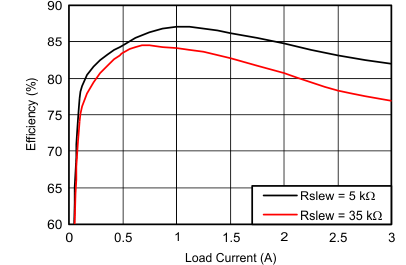V(VIN) = 12 V V(VReg) = 5 V f(SW) = 500 kHz L = 22 µH C4 (output) = 100 µF TA = 25ºC
Figure 1. FET Switching (Slow Slew Rate)V(VReg) = 5 V f(SW) = 500 kHz L = 22 µH C4 (output) = 100 µF Rslew = 5 kΩ TA = 25ºC
Figure 2. Fast Slew Rate on Switching FET

#### 6.8.2 Output Voltage Dropout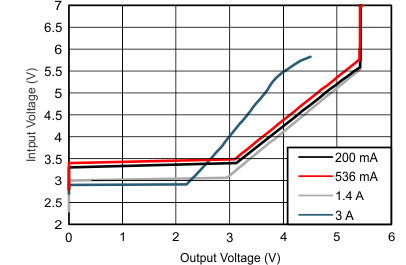V(VReg) = 5 V f(SW) = 500 kHz
Figure 3. Load Current > 100 mA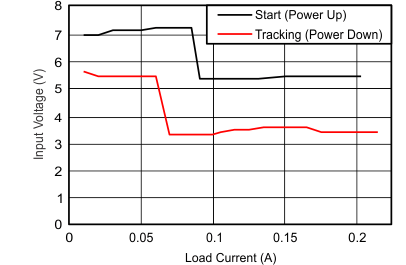V(VReg) = 5 V TA = 25ºC
Figure 5. Output-Voltage Tracking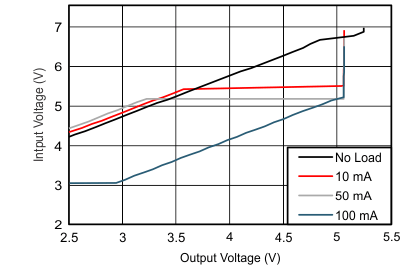V(VReg) = 5 V f(SW) = 500 kHz
Figure 4. Load Current < 100 mA

NOTE

Tracking: The input voltage at which the output voltage drops approximately –0.7 V of the regulated voltage or for low input voltages (tracking function) over the load range.

Start: The input voltage required to achieve 5-V regulation on power up with the stated load currents.

#### 6.8.3 Quiescent and Standby Current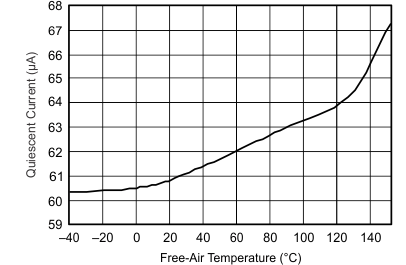V(VIN) = 12 V
Figure 6. LPM, Quiescent Current Variation With Temperature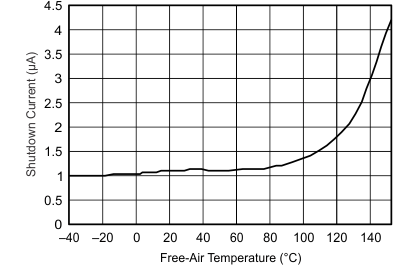V(VIN) = 12 V
Figure 8. Shutdown Current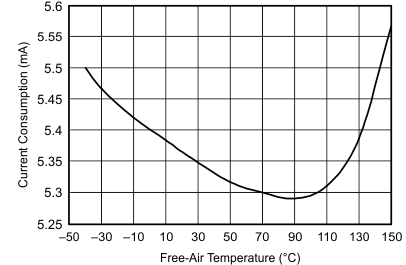EN = High V(VIN) = 12 V
Figure 10. Current Consumption vs TemperatureV(VIN) = 24 V
Figure 7. LPM, Quiescent Current Variation with Temperature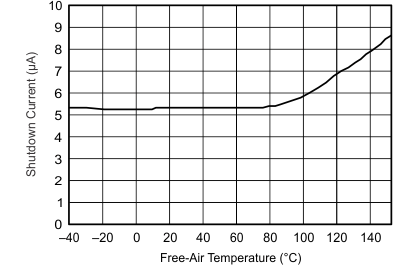V(VIN) = 24 V
Figure 9. Shutdown Current

#### 6.8.4 Reference Voltages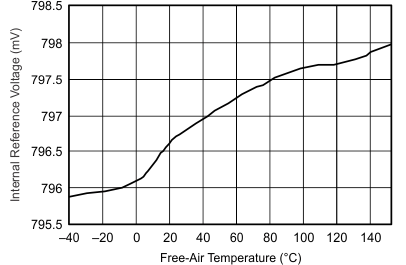V(VIN) = 12 V
Figure 11. Internal Reference Voltage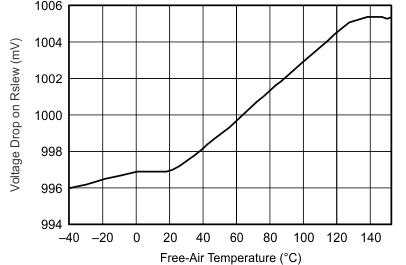Figure 12. Voltage Drop on Rslew for Current Reference